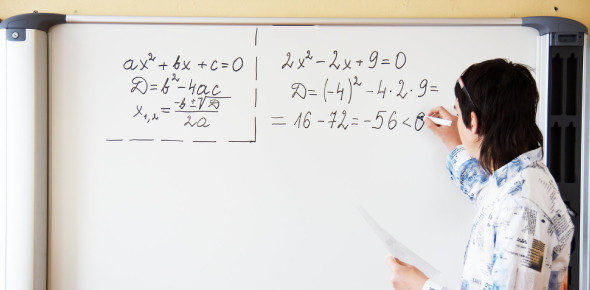# Grade 11 Standard And Factored Form Quiz

10 Questions | Attempts: 287SettingsDo you like practicing algebra? Take this grade 11 standard and factored form quiz to check your understanding of algebra. You can practice your algebra skills here and check out your results. This quiz is a perfect practice test if you want to know about your standard and factored-form knowledge but also your knowledge. So, you must go for this quiz and practice these math questions. All the best for a perfect score! Do share the quiz with other math experts.

• 1.
Which shows the quadratic function expressed in factored form? f(x) = 2x2 + 9x – 5
• A.

(2x + 5)(x – 1)

• B.

(2x – 1)(x + 5)

• C.

(2x + 1)(x + 5)

• D.

(2x – 1)(x – 5)

• 2.
The height of a crab h(t) in meters, dropped by a seagull to the rocks below, is given by the function h(t) = –5t2 + 20, where t is the time, in seconds, after it is released. What are the zeros of the function?
• A.

(–5, 0) and (20, 0)

• B.

(2, 0) and (0, 0)

• C.

(–2, 0) and (20, 0)

• D.

(–5, 0) and (4, 0)

• 3.
What is the axis of symmetry for the function –x2 + 7x + 30
• A.

X = –3

• B.

X = 3.5

• C.

X = 6.5

• D.

X = 10

• 4.
A volleyball is hit straight upward. The graph shows its height, h(t), in meters, at time t. Which is the function in a factored form that estimates the height of the volleyball at any given time?
• A.

X(x – 2)

• B.

–5x(x + 2)

• C.

–5(x – 2)

• D.

–5x(x – 2)

• 5.
What is the y–intercept of the quadratic function f(x) = (2x + 4)(x – 3)?
• A.

(0, –12)

• B.

(0, 3)

• C.

(0, –2)

• D.

(0, 12)

• 6.
A rocket is shot into the air. The height of the rocket is modeled by the function h(t) = –5t2 + 45t, where h(t) is the height in meters and t is the time in seconds. When will the rocket hit the ground?
• A.

4.5 sec

• B.

5 sec

• C.

9 sec

• D.

45 sec

• 7.
What are the coordinates of the vertex of f(x) = 2x2 – 6x – 20?
• A.

(–2, 5)

• B.

(1.5, –24.5)

• C.

(–2, –24)

• D.

(5, 0)

• 8.
A rock is thrown into the river from a bridge that is 20 meters high. The table shows its height, h(t), at time t. Determine a function, in factored form, that estimates the height of the rock at any given time.
• A.

5(x+1)(x–4)

• B.

–5(x–1)(x+4)

• C.

–5(x+0)(x+4)

• D.

–5(x+1)(x–4)

• 9.
Which is the graph of the function g(x) = (2x – 1)(x – 5)?
• A.

Graph A

• B.

Graph B

• C.

Graph C

• D.

Graph D

• 10.
A fountain shoots water from a muzzle at its top. The function f(x) = –5t2 + 10t + 15 describes the height of the water h(t) in meters, t seconds after it leaves the nozzle. What is the maximum height of the water spout?
• A.

1m

• B.

3m

• C.

15m

• D.

20m

## Related TopicsBack to top
×

Wait!
Here's an interesting quiz for you.